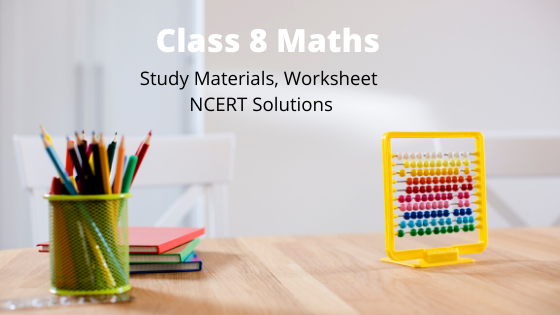# Class 8 Maths study Material & NCERT Solutions

•• Welcome to our class 8 Maths page. In this page you can find class 8 Maths ncert solutions , notes, assignments, worksheets etc. You can share the link to free Class 8 NCERT Science solutions with your classmates as all study material is free of cost.

• First read and understand the notes.
• Try to go through the solved examples of of the chapter you are reading
• Now it is the time to solve unsolved assignments and worksheets. You will find plenty of them here or on the respective chapter page.
All the best for better learning of your maths concepts. Hope we helped you when you were looking for class 8 Maths notes .

## Chapter 1:Rational Numbers

Notes & Worksheet
NCERT Solutions

## Chapter 2: Linear equation in One variable

Notes & Worksheet
NCERT Solutions

Notes & Worksheet
NCERT Solutions

## Chapter 5: Data Handling

Notes & Worksheet

## Chapter 6: Square and Square roots

Notes & Worksheet
NCERT Solutions

## Chapter 7: Cube and Cube roots

Notes & Worksheet
NCERT Solutions

## Chapter 8:Comparing quantities

Notes & Worksheet
NCERT Solutions

## Chapter 9: Algebraic Expressions and Identities

Notes & Worksheet
NCERT Solutions

## Chapter 11: Mensurations

Notes & Worksheet
NCERT Solutions

## Chapter 12: Exponents and Power

Notes & Worksheet
NCERT Solutions

## Chapter 13:Direct and Inverse proportion

Notes & Worksheet
NCERT Solutions

## Chapter 14:Factorisation

Notes & Worksheet
NCERT Solutions

## Chapter 15:Introduction to Graphs

Notes & Worksheet
NCERT Solutions

## Frequently asked Questions on CBSE Class 8 Maths

### How many chapters are there in Class 8 Maths?

There are total 16 chapters in Class 8 Maths. All the chapters are required for assessment

### What is the best books for Class 8 Maths?

Couple of book
(a)NCERT Class 8 Maths textbook
(b)NCERT Class 8 Exemplar
(c) RD Sharma or RS agarwal

### Which is the easiest chapter of class 8 Maths NCERT Text book?

Mensuration and Linear equations in one variable are the easiest chapter

### Is Class 8 Maths NCERT Text book Sufficient for CBSE Exams?

It is sufficient if you go through all the questions in the NCERT text book thoroughly and practice them all.However ti enhance your maths skill , you can go for NCERT exemplar and other books

### what is the best way to learn the concept in Class 8 Maths?

First go through all the Notes and solved examples and Then do the exercises. Practice Lot of questions. The simple Mantra is Practice .Practice and Practice